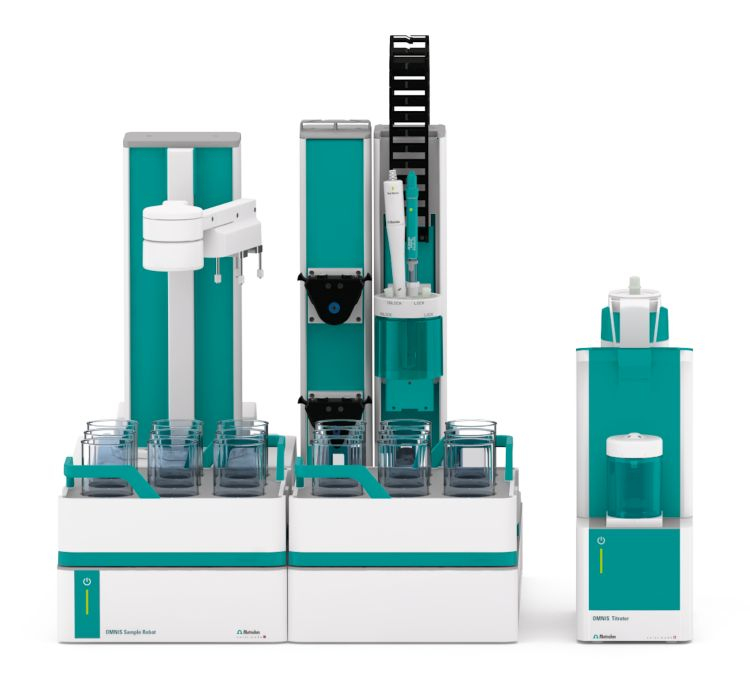You have been redirected to your local version of the requested page

Citric acid and oxalic acid are present in many products, such as foods or chemical solvents (e.g., decontamination solutions). Both acids are reducing agents, and citric acid is additionally a powerful antioxidant.

Both of these acids can be individually determined using titration. However, to determine their concentrations in mixtures, a content calculation is only possible with correction factors for each acid due to their mutual impact (buffer effect).

A fast and accurate determination of these acids in various mixtures by potentiometric titration using the dEcotrode plus and sodium hydroxide as titrant can be realized. This Application Note explains more about this fast and easy analysis with reliable, automated titration instruments from Metrohm.

The analysis is demonstrated on a mixture of citric acid and oxalic acid (β(citric acid) = 20 g/L and β(oxalic acid) = 20 g/L).

No sample preparation is required.Figure 1. OMNIS System consisting of an OMNIS Sample Robot S and an OMNIS Advanced Titrator.

The analyses are carried out fully automatically on a OMNIS Sample Robot S in combination with an OMNIS Advanced Titrator and the dEcotrode plus for indication.

The sample solution is transferred into a sample beaker and deionized water is added. The solution is titrated with standardized sodium hydroxide until after the second equivalence point. After each titration, the solution is aspirated and the electrode is then rinsed with deionized water.

Reproducible titration curves (see Figure 2) are obtained for all analyses. The first equivalence point corresponds to oxalic acid and the second to citric acid. However, the two obtained equivalence points influence each other due to the close pKa values of the acids (oxalic acid = 1.25 and 4.14, citric acid = 3.13, 4.76, and 6.39). Therefore, a correction factor is required for the titration. The correction factors used for this sample are 0.904 for citric acid, and 1.11 for oxalic acid.

The automated analysis leads to reproducible results with a RSD < 1.5% as shown in Table 1.

Figure 2. Titration curve of the determination of a mixture of citric and oxalic acid. The first equivalence point corresponds to oxalic acid, and the second to citric acid.
Table 1. Results of the determination of the mixture of β(citric acid) = 20 g/L and β(oxalic acid) = 20 g/L (n = 5).
Acid Mean value / (g/L) SD(abs) / (g/L) SD(rel) /%
Citric acid 19.68 0.26 1.3
Oxalic acid 19.59 0.14 0.7

As a non-linear-correlation between the correction factors and the ratio of citric acid and oxalic acid exists, it is recommended to determine the correction factors at the expected ratio of citric and oxalic acid using standard solutions.

However, this method provides an easy and fast way to determine the citric acid and oxalic acid content in mixtures by potentiometric titration. The determination of the factors can be done automatically using the OMNIS system.